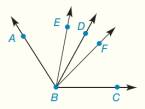Chapter 1.CT, Problem 23CT### Elementary Geometry for College St...

6th Edition
Daniel C. Alexander + 1 other
ISBN: 9781285195698

#### Solutions

Chapter
Section### Elementary Geometry for College St...

6th Edition
Daniel C. Alexander + 1 other
ISBN: 9781285195698
Textbook Problem
1 views

# Obtuse angle ABC is bisected by B D → and is trisected by B E → and B F → . If m ∠ E B D = 18 ° , find m ∠ A B C .________To determine

To find:

The value of mABC.

Explanation

Given:

The given figure is,

Obtuse angle ABC is bisected by BD and is trisected by BE and BF and mEBD=18°.

Calculation:

Let mABC be x°.

Then, mABD=mBCD=(x2),

And, mABE=mEBF=mCBF=(x3).

Now,

### Still sussing out bartleby?

Check out a sample textbook solution.

See a sample solution

#### The Solution to Your Study Problems

Bartleby provides explanations to thousands of textbook problems written by our experts, many with advanced degrees!

Get Started

#### Find more solutions based on key concepts